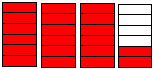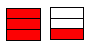Name: ___________________Date:___________________

 Email us to get an instant 20% discount on highly effective K-12 Math & English kwizNET Programs!

Math Word Problems - GED, PSAT, SAT, ACT, GRE Preparation5.20 Mixed Numbers Using Pictures

 Mixed fractions are made up of a whole number and a fraction. Example:In the figure two of them are completely filled and the third is 3 out of 5 Answer: 2 3/5 Directions: Write the corresponding mixed fractions. Also write at least 5 examples of your own.
 Q 1: Express the figure as a mixed fraction.2 1/12 1/32 1/2 Q 2: Express the figure as a mixed fraction.3 1/63 4/63 2/6 Q 3: Express the figure as a mixed fraction.2 2/63 4/61 4/6 Q 4: Express the figure as a mixed fraction.2 2/52 4/52 3/5 Q 5: Express the figure as a mixed fraction.2 3/41 3/42 3/3 Q 6: Express the figure as a mixed fraction.2 1/31 1/31 2/3 Question 7: This question is available to subscribers only! Question 8: This question is available to subscribers only!